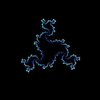# lambda calculus proof help - General

Welcome to the Functional Programming Zulip Chat Archive. You can join the chat here.Ah - I guess you can use transitivity of $r^\star$ on $c \to_r b \to_r^\star d$ to create $c \to_r^\star e$?thinking about it more, I don't understand why that $e$ is even there in the first place. $a\to_{r^*}d$ and $c\to_{r^*}d$, that should be QED :thinking:It exists in the second branch of the proof because it completes the diamond (d <- b -> c) but in the third branch the arrows are not in the right direction (d <- b <- c).@Mason Mackaman Have you tried emailing Helmut? Not only would he appreciate the tip-off, you'd also get what should've been Part 3 of the proof.@Kim-Ee Yeoh oh do they list an email? I've found 3 mistakes so far and I'm only on page 18. regarding the proof, I think I already know the correction.The email is on the very first page of the document, albeit in a smaller font: Helmut Brandl (firstname dot lastname at gmx dot net)